Impact Factor 2.638 | CiteScore 2.3
More on impact ›

# Frontiers in Physics## Brief Research Report ARTICLE

Front. Phys., 05 November 2020 | https://doi.org/10.3389/fphy.2020.555573

# Some Properties of Relative Bi-(Int-)Γ-Hyperideals in Ordered Γ-SemihypergroupsYongsheng Rao1,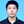Peng Xu1,2,Zehui Shao1,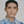Saeed Kosari1* andSaber Omidi3
• 1Institute of Computing Science and Technology, Guangzhou University, Guangzhou, China
• 2School of Computer Science of Information Technology, Qiannan Normal University for Nationalities, Duyun, China
• 3Department of Education in Tehran, Tehran, Iran

In this article, we study the concept of relative bi-$\text{Γ}$-hyperideals ($\mathcal{J}$-bi-$\text{Γ}$-hyperideals) in ordered $\text{Γ}$-semihypergroups and present some related examples of this concept. Especially, characterization of $\mathcal{J}$-simple ordered $\text{Γ}$-semihypergroups in terms of $\mathcal{J}$-bi-$\text{Γ}$-hyperideals is given. Furthermore, we define the notion of $\mathcal{J}$-(bi-)int-$\text{Γ}$-hyperideals in ordered $\text{Γ}$-semihypergroups and investigate its related properties. We show that $\mathcal{J}$-int-$\text{Γ}$-hyperideals and $\mathcal{J}$-$\text{Γ}$-hyperideals coincide in a $\mathcal{J}$-regular ordered $\text{Γ}$-semihypergroup.

## 1. Introduction

One of the motivations for the study of hyperstructures comes from biological inheritance and physical phenomenon as the nuclear fission. Another motivation for the study of hyperstructures comes from chemical reactions and redox reactions. Dehghan Nezhad et al.  provided a physical example of hyperstructures associated with the elementary particle physics: leptons. As we know, the Higgs boson is an elementary particle in the standard model of particle physics. In , it is shown that the leptons and gauge bosons along with the interactions between their members construct an ${H}_{\nu }$-structure. Yaqoob et al.  studied some properties of (fuzzy) $\text{Γ}$-hyperideals in involution $\text{Γ}$-semihypergroups. In , the concepts of uni-soft $\text{Γ}$-hyperideals and uni-soft interior $\text{Γ}$-hyperideals of ordered $\text{Γ}$-semihypergroups are investigated.

Algebraic hyperstructures are a suitable generalization of classical algebraic structures. In a classical algebraic structure, the composition of two elements is an element, while in an algebraic hyperstructure, the composition of two elements is a set. The concept of hyperstructures was first introduced by Marty  at the eighth congress of Scandinavian Mathematicians in 1934. Nowadays, we can easily find well-written books for the introduction to hyperstructures, which include Corsini , Corsini and Leoreanu , Davvaz , Davvaz and Leoreanu-Fotea , Davvaz and Vougiouklis , and Vougiouklis . For the information about hyper rings, we refer the reader to Ref. 9.

The study of ordered semihypergroups began with the work of Heidari and Davvaz . In 2015, Davvaz et al.  discussed the notion of a pseudo-order in an ordered semihypergroup. The focus of the study was to find out if there is a relationship between ordered semihypergroups and ordered semigroups by using pseudo-orders. In 2016, Gu and Tang  answered to the open problem given by Davvaz et al. . In , Tipachot and Pibaljommee introduced the concept of fuzzy interior hyperideals on ordered semihypergroups. Recently, Mahboob et al.  studied the concept of $\left(m,n\right)$-hyperideals on ordered semihypergroups. Recall, from Ref. 12, that an ordered semihypergroup $\left(S,\circ ,\le \right)$ is a semihypergroup $\left(S\right)$ together with a (partial) order relation $\le$ that is compatible with the hyperoperation $\circ$, meaning that, for any $x,y,z\in S$,

$x≤y⇒z∘x≤z∘y and x∘z≤y∘z.$

Here, $A\le B$ means that for any $a\in A$, there exists $b\in B$ such that $a\le b$, for all nonempty subsets A and B of S.

Good and Hughes  introduced the notion of bi-ideals of a semigroup as early as 1952. In 1962, Wallace  introduced the notion of relative ideals ($\mathcal{J}$-ideals) on semigroups. In 1967, Hrmová  generalized the notion of $\mathcal{J}$-ideal by introducing the notion of a $\left({\mathcal{J}}_{1},{\mathcal{J}}_{2}\right)$-ideal of a semigroup S. Recently, Khan and Ali  introduced the concept of relative bi-ideals in ordered semigroups. The notion of $\text{Γ}$-semigroup was introduced by Sen and Saha  in 1986, which is a generalization of semigroups. In 2011, Anvariyeh et al.  introduced the notion of $\text{Γ}$-hyperideal of a $\text{Γ}$-semihypergroup. Later on, Yaqoob and Aslam  studied prime $\left(m,n\right)$ bi-$\text{Γ}$-hyperideals of $\text{Γ}$-semihypergroups. Omidi et al. [24, 25] discussed some important properties of bi-$\text{Γ}$-hyperideals of an ordered $\text{Γ}$-semihypergroup. Bi-$\text{Γ}$-hyperideal is a special case of $\left(m,n\right)$-$\text{Γ}$-hyperideal . In 2017, Tang et al.  considered and proved some theorems on fuzzy interior $\text{Γ}$-hyperideals in ordered $\text{Γ}$-semihypergroups. Pseudo-orders are the bridge between ordered $\text{Γ}$-semigroups and ordered $\text{Γ}$-semihypergroups, see Ref. 28.

After an introduction, in Section 2, we introduce some notation and terminologies. Section 2 aims for summarizing the fundamental materials on ordered $\text{Γ}$-semihypergroups. Section 3 is devoted to the study of relative $\text{Γ}$-hyperideals ($\mathcal{J}$-$\text{Γ}$-hyperideals) and relative bi-$\text{Γ}$-hyperideals ($\mathcal{J}$-bi-$\text{Γ}$-hyperideals) of an ordered $\text{Γ}$-semihypergroup. In this section, our main results are stated and proved. $\mathcal{J}$-simple ordered $\text{Γ}$-semihypergroups are characterized by using the notions of $\mathcal{J}$-$\text{Γ}$-hyperideals and $\mathcal{J}$-bi-$\text{Γ}$-hyperideals. Finally, in Section 4, the notion of $\mathcal{J}$-(bi-)int-$\text{Γ}$-hyperideals ($\mathcal{J}$-(bi-)interior-$\text{Γ}$-hyperideals) are studied and their related properties are discussed. It is shown that, in $\mathcal{J}$-regular ordered $\text{Γ}$-semihypergroups the $\mathcal{J}$-$\text{Γ}$-hyperideals and the $\mathcal{J}$-int-$\text{Γ}$-hyperideals coincide.

## 2. Preliminaries

Let S and $\text{Γ}$ be two nonempty sets. Then, S is called a $\text{Γ}$-semihypergroup  if every $\gamma \in \text{Γ}$ is a hyperoperation on S, i.e., $x\gamma y\subseteq S$, for every $x,y\in S$, $\alpha ,\beta \in \text{Γ}$, and $x,y,z\in S$, we have

$xα(yβz)=(xαy)βz.$

If every $\gamma \in \Gamma$ is an operation, then S is a $\text{Γ}$-semigroup. Let A and B be two nonempty subsets of S. We define

$AγB=∪{aγb | a∈A,b∈B}.$

Also,

$AΓB=∪{aγb | a∈A,b∈B and γ∈Γ}=∪γ∈ΓAγB.$

In the following, we recall the notion of an ordered $\text{Γ}$-semihypergroup, and then we present basic definitions and notations, which we will need in this article. Throughout this article, unless otherwise specified, S is always an ordered $\text{Γ}$-semihypergroup $\left(S,\text{Γ},\le \right)$.

Definition 2.1 (see ). An algebraic hyperstructure $\left(S,\text{Γ},\le \right)$ is called an ordered $\text{Γ}$-semihypergroup if $\left(S,\text{Γ}\right)$ is a $\text{Γ}$-semihypergroup and $\left(S\right)$ is a partially ordered set such that for any $x,y,z\in S$ and $\gamma \in \text{Γ}$, $x\le y$ implies $z\gamma x\le z\gamma y$ and $x\gamma z\le y\gamma z$. Here, if A and B are two nonempty subsets of S, then we say that $A\le B$ if, for every $a\in A$, there exists $b\in B$ such that $a\le b$.

Let S be an ordered $\text{Γ}$-semihypergroup. By a sub $\text{Γ}$-semihypergroup of S, we mean a nonempty subset A of S such that $a\gamma b\subseteq A$ for all $a,b\in A$ and $\gamma \in \text{Γ}$. A nonempty subset A of S is called idempotent if $A=\left(A\Gamma A\right]$.

Example 1. (See Ref. 25.) Let $\left(S,\circ ,\le \right)$ be an ordered semihypergroup and $\text{Γ}$ a nonempty set. We define $a\gamma b=a\circ b$ for every $a,b\in S$ and $\gamma \in \text{Γ}$. Then, $\left(S,\text{Γ},\le \right)$ is an ordered $\text{Γ}$-semihypergroup.

Let $\mathcal{J}$ be a nonempty subset of an ordered $\text{Γ}$-semihypergroup $\left(S,\text{Γ},\le \right)$. If H is a nonempty subset of $\mathcal{J}$, then we define

$(H]J:={j∈J | j≤h for some h∈H}.$

Note that if $\mathcal{J}=S$, then we define

$(H]:={x∈S | x≤h for some h∈H}.$

If A and B are nonempty subsets of S, then we have

(1) $A\subseteq {\left(A\right]}_{\mathcal{J}}$ for all $A\subseteq \mathcal{J}$

(2) ${\left({\left(A\right]}_{\mathcal{J}}\right]}_{\mathcal{J}}={\left(A\right]}_{\mathcal{J}}$

(1) If $A\subseteq B\subseteq \mathcal{J}$, then ${\left(A\right]}_{\mathcal{J}}\subseteq {\left(B\right]}_{\mathcal{J}}$

(2) ${\left(A\right]}_{\mathcal{J}}\text{Γ}{\left(B\right]}_{\mathcal{J}}\subseteq {\left(A\text{Γ}B\right]}_{\mathcal{J}}$

An element a of an ordered $\text{Γ}$-semihypergroup $\left(S,\Gamma ,\le \right)$ is regular  if there exist $x\in S$ and $\alpha ,\beta \in \Gamma$ such that $a\le a\alpha x\beta a$. This is equivalent to saying that $a\in \left(a\Gamma S\Gamma a\right]$, for each $a\in S$. An ordered $\text{Γ}$-semihypergroup S is said to be regular if every element of S is a regular element.

Definition 2.2. Let $\left(S,\Gamma ,\le \right)$ be an ordered $\text{Γ}$-semihypergroup. A nonempty subset A of S is called a left (resp. right) $\text{Γ}$-hyperideal  of S if it satisfies the following conditions:

(1) $S\Gamma A\subseteq A$ (resp. $A\Gamma S\subseteq A$)

(2) If $x\in A$, $y\in S$, and $y\le x$, then $y\in A$

If A is both a left $\text{Γ}$-hyperideal and a right $\text{Γ}$-hyperideal of S, then it is called a $\text{Γ}$-hyperideal (or two-sided $\text{Γ}$-hyperideal) of S.

## 3. Basic Properties of Relative Bi-$\text{Γ}$-Hyperideals ($\mathcal{J}$-Bi-$\text{Γ}$-Hyperideals)

Let $\left(S,\Gamma ,\le \right)$ be an ordered $\text{Γ}$-semihypergroup and $\mathrm{ℐ}$ and $\mathcal{J}$ be the nonempty subsets of S. Then, $\mathrm{ℐ}$ is called a left $\mathcal{J}$-$\text{Γ}$-hyperideal of S if it satisfies the following conditions:

(1) $\mathcal{J}\Gamma \mathrm{ℐ}\subseteq \mathrm{ℐ}$

(2) When $x\in \mathcal{J}$ and $y\in \mathrm{ℐ}$ such that $x\le y$, it implies that $x\in \mathrm{ℐ}$

A right $\mathcal{J}$-$\text{Γ}$-hyperideal of an ordered $\text{Γ}$-semihypergroup S is defined in a similar way. By two-sided $\mathcal{J}$-$\text{Γ}$-hyperideal or simply $\mathcal{J}$-$\text{Γ}$-hyperideal, we mean a nonempty subset of S which is both left and right $\mathcal{J}$-$\text{Γ}$-hyperideal of S.

Definition 3.1. Let ${\mathcal{J}}_{1}$ and ${\mathcal{J}}_{2}$ be the nonempty subsets of S. A nonempty subset $\mathrm{ℐ}$ of S is said to be an $\left({\mathcal{J}}_{1},{\mathcal{J}}_{2}\right)$-$\text{Γ}$-hyperideal of S if it satisfies the following conditions:

(1) ${\mathcal{J}}_{1}\Gamma \mathrm{ℐ}\subseteq \mathrm{ℐ}$ and $\mathrm{ℐ}\Gamma {\mathcal{J}}_{2}\subseteq \mathrm{ℐ}$

(2) When $x\in {\mathcal{J}}_{1}\cup {\mathcal{J}}_{2}$ and $y\in \mathrm{ℐ}$ such that $x\le y$, it implies that $x\in \mathrm{ℐ}$

Example 2. Let $S=\left\{a,b,c,d,e,f\right\}$ and $\Gamma =\left\{\gamma ,\beta \right\}$ be the sets of binary hyperoperations defined as follows: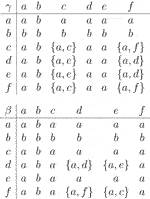Then, S is a $\text{Γ}$-semihypergroup . We have $\left(S,\text{Γ},\le \right)$ as an ordered $\text{Γ}$-semihypergroup, where the order relation $\le$ is defined by

$≤:={(a,a),(b,a),(b,b),(b,c),(b,f),(c,c),(d,d),(e,e),(f,f)}.$

The covering relation and the figure of S are given by

$≺={(b,a),(b,c),(b,f)}.$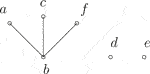Let ${\mathcal{J}}_{1}=\left\{a,b,c\right\},{\mathcal{J}}_{2}=\left\{b,d,f\right\}$, and $\mathrm{ℐ}=\left\{a,b,f\right\}$. One can check that $\mathrm{ℐ}$ is a $\left({\mathcal{J}}_{1},{\mathcal{J}}_{2}\right)$-$\text{Γ}$-hyperideal of S.Here are some elementary properties of these concepts.

Lemma 3.2. Let $\left(S,\Gamma ,\le \right)$ be an ordered $\Gamma$-semihypergroup. If $\mathcal{J}$ is a sub $\text{Γ}$-semihypergroup of S, then ${\left(\mathcal{J}\Gamma a\Gamma \mathcal{J}\right]}_{\mathcal{J}}$ is a $\mathcal{J}$-$\text{Γ}$-hyperideal of S for each $a\in S$.

Proof. Let $a\in S$. We show that ${\left(\mathcal{J}\Gamma a\Gamma \mathcal{J}\right]}_{\mathcal{J}}$ is a $\mathcal{J}$-$\text{Γ}$-hyperideal of S. We have

$JΓ(JΓaΓJ]J=(J]JΓ(JΓaΓJ]J⊆(JΓ(JΓaΓJ)]J=(JΓJ(ΓaΓJ)]J⊆(JΓaΓJ]J.$

Similarly, we have ${\left(\mathcal{J}\text{Γ}a\text{Γ}\mathcal{J}\right]}_{\mathcal{J}}\text{Γ}\mathcal{J}\subseteq {\left(\mathcal{J}\text{Γ}a\text{Γ}\mathcal{J}\right]}_{\mathcal{J}}$. Now, let $x\in \mathcal{J}$ and $y\in {\left(\mathcal{J}\Gamma a\Gamma \mathcal{J}\right]}_{\mathcal{J}}$ such that $x\le y$. Then, $x\le y\le z$ for some $z\in \mathcal{J}\Gamma a\Gamma \mathcal{J}$. Hence, $x\le z$ and so $x\in {\left(\mathcal{J}\text{Γ}a\text{Γ}\mathcal{J}\right]}_{\mathcal{J}}$. Therefore, ${\left(\mathcal{J}\Gamma a\Gamma \mathcal{J}\right]}_{\mathcal{J}}$ is a $\mathcal{J}$-$\text{Γ}$-hyperideal of S.

Theorem 3.3. Let I be a left $\text{Γ}$-hyperideal of an ordered $\text{Γ}$-semihypergroup $\left(S,\Gamma ,\le \right)$ and $\varnothing \ne \mathcal{J}\subseteq S$ such that $I\subseteq \mathcal{J}$. If M is an idempotent left $\mathcal{J}$-$\text{Γ}$-hyperideal of I, then M is a left $\text{Γ}$-hyperideal of S.

Proof. Clearly, I is an ordered sub-$\text{Γ}$-semihypergroup of S. We have

$SΓM=SΓ(MΓM]=(S]Γ(MΓM]⊆(SΓ(MΓM)]=((SΓM)ΓM]⊆((SΓI)ΓM]⊆(IΓM]⊆(JΓM]⊆(M]J=M.$

If $m\in M\subseteq I$ and $x\in S$ such that $x\le m$, then we have $x\in I\subseteq \mathcal{J}$. Since M is a left $\mathcal{J}$-$\text{Γ}$-hyperideal of I, it follows that $x\in M$. Hence, M is a left $\text{Γ}$-hyperideal of S. ∎

Theorem 3.4. Let I be a $\text{Γ}$-hyperideal of a regular ordered $\text{Γ}$-semihypergroup $\left(S,\text{Γ},\le \right)$ and ${\mathcal{J}}_{1}$, ${\mathcal{J}}_{2}\subseteq S$ such that $I\subseteq {\mathcal{J}}_{1}\cap {\mathcal{J}}_{2}$. Then, any $\left({\mathcal{J}}_{1},{\mathcal{J}}_{2}\right)$-$\text{Γ}$-hyperideal of I is a $\text{Γ}$-hyperideal of S.

Proof. Let I be a $\text{Γ}$-hyperideal of S. Then, $I\Gamma I\subseteq S\Gamma I\subseteq I$. So, I is an ordered sub-$\text{Γ}$-semihypergroup of S. Let A$\left(A\subseteq I\right)$ be a $\left({\mathcal{J}}_{1},{\mathcal{J}}_{2}\right)$-$\text{Γ}$-hyperideal of I. We prove that A is a $\text{Γ}$-hyperideal of S. Let $i\in I\subseteq S$. Then, there exist $x\in S$ and $\alpha ,\beta \in \Gamma$ such that

$i≤iαxβi≤iαxβ(iαxβi)=iα(xβiαx)βi.$

Since I is a $\text{Γ}$-hyperideal of S, it follows that

$xβiαx⊆(SΓI)ΓS⊆IΓS⊆I.$

Hence, $i\le t$, for some $t\in i\alpha \left(x\beta i\alpha x\right)\beta i\subseteq i\Gamma I\Gamma i$. This means that $i\in {\left(i\text{Γ}I\text{Γ}i\right]}_{I}$. Therefore, I is a regular ordered sub-$\text{Γ}$-semihypergroup of S.

Let $a\in A$$\left(A\subseteq I\right)$ and $s\in S$. Then, $a\gamma s\subseteq I$ where $\gamma \in \text{Γ}$. Now, suppose that $v\in a\gamma s\subseteq I$. Then, there exist $y\in I$ and $\lambda ,\mu \in \text{Γ}$ such that

$v≤vλyμv⊆(aγs)λyμ(aγs)⊆AΓ(SΓIΓI)ΓS⊆AΓIΓS⊆AΓI⊆AΓ(J1∩J2)⊆AΓJ2⊆A.$

Similarly, we have $s\gamma a\subseteq A$.

If $u\in A\subseteq I$ and $v\in S$ such that $v\le u$, then we have $u\in I\subseteq {\mathcal{J}}_{1}\cap {\mathcal{J}}_{2}\subseteq {\mathcal{J}}_{1}\subseteq {\mathcal{J}}_{1}\cup {\mathcal{J}}_{2}$. Since A is a $\left({\mathcal{J}}_{1},{\mathcal{J}}_{2}\right)$-$\text{Γ}$-hyperideal of I, it follows that $v\in A$. Hence, A is a $\text{Γ}$-hyperideal of S.

Let a be an element of an ordered $\text{Γ}$-semihypergroup $\left(S,\text{Γ},\le \right)$. We denote by ${L}_{1}\left(a\right)$ (resp. ${R}_{2}\left(a\right)$, ${I}_{\mathcal{J}}\left(a\right)$) the left (resp. right, two-sided) relative $\text{Γ}$-hyperideal of S generated by a. The intersection of all $\left({\mathcal{J}}_{1},{\mathcal{J}}_{2}\right)$-$\text{Γ}$-hyperideals of S containing the element a is denoted by ${I}_{\mathcal{J}}\left(a\right)$, where $\mathcal{J}={\mathcal{J}}_{1}\cup {\mathcal{J}}_{2}$.

Lemma 3.5. Let a be an element of an ordered $\text{Γ}$-semihypergroup $\left(S,\Gamma ,\le \right)$ and ${\mathcal{J}}_{1}$ and ${\mathcal{J}}_{2}$ be two sub-$\text{Γ}$-semihypergroups of S. Then,

(1) ${L}_{1}\left(a\right)={\left(a\cup {\mathcal{J}}_{1}\Gamma a\right]}_{{\mathcal{J}}_{1}}$

(2) ${R}_{2}\left(a\right)={\left(a\cup a\Gamma {\mathcal{J}}_{2}\right]}_{{\mathcal{J}}_{2}}$

(3) ${I}_{\mathcal{J}}\left(a\right)={\left(a\cup {\mathcal{J}}_{1}\Gamma a\cup a\Gamma {\mathcal{J}}_{2}\cup {\mathcal{J}}_{1}\Gamma a\Gamma {\mathcal{J}}_{2}\right]}_{\mathcal{J}}$, where $\mathcal{J}={\mathcal{J}}_{1}\cup {\mathcal{J}}_{2}$

Proof. Since $a\in {L}_{1}\left(a\right)$ and ${\mathcal{J}}_{1}\Gamma a\subseteq {L}_{1}\left(a\right)$, it follows that ${\left(a\cup {\mathcal{J}}_{1}\Gamma a\right]}_{{\mathcal{J}}_{1}}\subseteq {L}_{1}\left(a\right)$. We have

$J1Γ(a∪J1Γa]J1⊆(J1]J1Γ(a∪J1Γa]J1⊆(J1Γ(a∪J1Γa)]J1⊆(J1Γa]J1⊆(a∪J1Γa]J1.$

On the contrary, we have ${\left({L}_{1}\left(a\right)\right]}_{{\mathcal{J}}_{1}}={L}_{1}\left(a\right)$. Thus, ${L}_{1}\left(a\right)={\left(a\cup {\mathcal{J}}_{1}\Gamma a\right]}_{{\mathcal{J}}_{1}}$ is a left ${\mathcal{J}}_{1}$-$\text{Γ}$-hyperideal of S containing a. This means that ${L}_{1}\left(a\right)\subseteq {\left(a\cup {\mathcal{J}}_{1}\Gamma a\right]}_{{\mathcal{J}}_{1}}$.

Now, we show that ${L}_{1}\left(a\right)$ is the smallest left ${\mathcal{J}}_{1}$-$\text{Γ}$-hyperideal of S containing a. Suppose that A is a left ${\mathcal{J}}_{1}$-$\text{Γ}$-hyperideal of S containing a. We have

$L1(a)=(a∪J1Γa]J1⊆(A∪J1ΓA]J1⊆(A]J1⊆A.$

This proves that (1) holds. Conditions (2) and (3) are proved similarly.

Let $\left(S,\Gamma ,\le \right)$ be an ordered $\text{Γ}$-semihypergroup and ${\mathcal{J}}_{1}$ and ${\mathcal{J}}_{2}$ be nonempty subsets of S. Then, S is said to be left ${\mathcal{J}}_{1}$-simple if it has no proper left ${\mathcal{J}}_{1}$-$\text{Γ}$-hyperideal. In the same way, we can define a right ${\mathcal{J}}_{2}$-simple ordered $\text{Γ}$-semihypergroup. If S is a left ${\mathcal{J}}_{1}$-simple and right ${\mathcal{J}}_{2}$-simple) ordered $\text{Γ}$-semihypergroup, then S is a $\left({\mathcal{J}}_{1}$, ${\mathcal{J}}_{2}\right)$-simple ordered $\text{Γ}$-semihypergroup.

Lemma 3.6. Let $\left(S,\Gamma ,\le \right)$ be an ordered $\text{Γ}$-semihypergroup and ${\mathcal{J}}_{1}$ and ${\mathcal{J}}_{2}$ be sub-$\text{Γ}$-semihypergroups of S such that $\mathcal{J}={\mathcal{J}}_{1}\cup {\mathcal{J}}_{2}$. Then, the following assertions hold:

(1) S is left ${\mathcal{J}}_{1}$-simple if and only if ${\left({\mathcal{J}}_{1}\text{Γ}a\right]}_{\mathcal{J}}=S$ for each $a\in S$

(2) S is right ${\mathcal{J}}_{2}$-simple if and only if ${\left(a\text{Γ}{\mathcal{J}}_{2}\right]}_{\mathcal{J}}=S$ for each $a\in S$

(3) S is (${\mathcal{J}}_{1}$, ${\mathcal{J}}_{2}$)-simple if and only if ${\left({\mathcal{J}}_{1}\Gamma a\Gamma {\mathcal{J}}_{2}\right]}_{\mathcal{J}}=S$ for each $a\in S$

Proof. The proof is straightforward.

We continue this section with the following definition.

Definition 3.7. Let $\left(S,\Gamma ,\le \right)$ be an ordered $\text{Γ}$-semihypergroup and $\mathcal{J}$ a nonempty subset of S. A sub-$\text{Γ}$-semihypergroup $\mathrm{ℬ}$ of S is called a relative bi-$\text{Γ}$-hyperideal ($\mathcal{J}$-bi-$\text{Γ}$-hyperideal) of S if the following conditions hold:

(1) $\mathrm{ℬ}\Gamma \mathcal{J}\Gamma \mathrm{ℬ}\subseteq \mathrm{ℬ}$

(2) When $a\in \mathcal{J}$ and $b\in \mathrm{ℬ}$ such that $a\le b$, it implies that $a\in \mathrm{ℬ}$

Example 3. We come back to Example 2 and consider ordered $\text{Γ}$-semihypergroup $\left(S,\text{Γ},\le \right)$. Let $\mathrm{ℬ}=\left\{a,b,c\right\}$ and $\mathcal{J}=\left\{b,f\right\}$. It is a routine matter to verify that $\mathrm{ℬ}$ is a $\mathcal{J}$-bi-$\text{Γ}$-hyperideal of S.

Lemma 3.8. The intersection of any family of $\mathcal{J}$-bi-$\text{Γ}$-hyperideals of an ordered $\text{Γ}$-semihypergroup $\left(S,\text{Γ},\le \right)$ is a $\mathcal{J}$-bi-$\text{Γ}$-hyperideal of S.

Proof. This proof is straightforward.

Let $\left(S,\text{Γ},\le \right)$ be an ordered $\text{Γ}$-semihypergroup and $\mathcal{J}$ be any nonempty subset of S. Then, S is said to be $\mathcal{J}$-regular if, for each $j\in \mathcal{J}$, there exist $x\in \mathcal{J}$ and $\alpha ,\beta \in \text{Γ}$ such that $j\le j\alpha x\beta j$.

Theorem 3.9. Let $\left(S,\text{Γ},\le \right)$ be an ordered $\text{Γ}$-semihypergroup and $\mathcal{J}$ a sub-$\text{Γ}$-semihypergroup of S. Then, the following assertions are equivalent:

(1) S is $\mathcal{J}$-regular

(2) $\mathrm{ℬ}={\left(\mathrm{ℬ}\text{Γ}\mathcal{J}\text{Γ}\mathrm{ℬ}\right]}_{\mathcal{J}}$ for every $\mathcal{J}$-bi-$\text{Γ}$-hyperideal $\mathrm{ℬ}$$\left(\subseteq \mathcal{J}\right)$ of S

Proof. $\left(1\right)⇒\left(2\right)$ Assume that (1) holds. Since $\mathrm{ℬ}$ is a $\mathcal{J}$-bi-$\text{Γ}$-hyperideal of S, we get $\mathrm{ℬ}\text{Γ}\mathcal{J}\text{Γ}\mathrm{ℬ}\subseteq \mathrm{ℬ}$. Thus, ${\left(\mathrm{ℬ}\text{Γ}\mathcal{J}\text{Γ}\mathrm{ℬ}\right]}_{\mathcal{J}}\subseteq {\left(\mathrm{ℬ}\right]}_{\mathcal{J}}=\mathrm{ℬ}$. Now, let $b\in \mathrm{ℬ}$$\left(\subseteq \mathcal{J}\right)$. Since S is $\mathcal{J}$-regular, there exist $x\in \mathcal{J}$ and $\alpha ,\beta \in \text{Γ}$ such that

$b≤bαxβb⊆ℬΓJΓℬ.$

Hence, $b\in {\left(\mathrm{ℬ}\Gamma \mathcal{J}\Gamma \mathrm{ℬ}\right]}_{\mathcal{J}}$ and so $\mathrm{ℬ}\subseteq {\left(\mathrm{ℬ}\text{Γ}\mathcal{J}\text{Γ}\mathrm{ℬ}\right]}_{\mathcal{J}}$. Therefore, $\mathrm{ℬ}={\left(\mathrm{ℬ}\text{Γ}\mathcal{J}\text{Γ}\mathrm{ℬ}\right]}_{\mathcal{J}}$.$\left(2\right)⇒\left(1\right)$ Let $\mathrm{ℛ}$ be a right $\mathcal{J}$-$\text{Γ}$-hyperideal and $\mathrm{ℒ}$ a left $\mathcal{J}$-$\text{Γ}$-hyperideal of S. By routine checking, we can easily verify that $\mathrm{ℛ}$ and $\mathrm{ℒ}$ are $\mathcal{J}$-bi-$\text{Γ}$-hyperideals of S. By Lemma 3.8, $\mathrm{ℛ}\cap \mathrm{ℒ}$ is a $\mathcal{J}$-bi-$\text{Γ}$-hyperideal of S. By hypothesis, we have

$ℛ∪ℒ=((ℛ∪​​ℒ)ΓJΓ(ℛ∪​​ℒ)]J⊆(ℛΓJΓℒ]J⊆(ℛΓℒ]J.$

Let $a\in \mathcal{J}$. Since $a\in {R}_{2}\left(a\right)$ and $a\in {L}_{1}\left(a\right)$, it follows that $a\in {R}_{2}\left(a\right)\cup {L}_{1}\left(a\right)$. By (1) and (2) of Lemma 3.5, we have

$a∈(R2(a)ΓL1(a)]J=((a∪aΓJ]JΓ(a∪JΓa]J]J=((a∪​​aΓJ)Γ(a∪​​JΓa)]J⊆(aΓa∪​​aΓJΓa]J.$

Then, $a\le w$, for some $w\in a\Gamma a\cup a\Gamma \mathcal{J}\Gamma a$. If $w\in a\Gamma a$, then $a\le a\gamma a\le a\gamma \left(a\gamma a\right)$. So, $a\in {\left(a\Gamma \mathcal{J}\Gamma a\right]}_{\mathcal{J}}$. Therefore, S is $\mathcal{J}$-regular. If $w\in a\Gamma \mathcal{J}\Gamma a$, then $a\le a\delta x\lambda a$, for some $x\in \mathcal{J}$ and $\delta ,\lambda \in \Gamma$. Thus, $a\in {\left(a\Gamma \mathcal{J}\Gamma a\right]}_{\mathcal{J}}$. Therefore, S is $\mathcal{J}$-regular.

Definition 3.10. Let $\left(S,\Gamma ,\le \right)$ be an ordered $\Gamma$-semihypergroup and ${\mathcal{J}}_{1}$ and ${\mathcal{J}}_{2}$ be the nonempty subsets of S. A sub-$\text{Γ}$-semihypergroup $\mathrm{ℬ}$ of S is said to be a $\left({\mathcal{J}}_{1},{\mathcal{J}}_{2}\right)$-bi-$\text{Γ}$-hyperideal of S if it satisfies the following conditions:

(1) $\mathrm{ℬ}\Gamma \left({\mathcal{J}}_{1}\cup {\mathcal{J}}_{2}\right)\Gamma \mathrm{ℬ}\subseteq \mathrm{ℬ}$

(2) When $a\in {\mathcal{J}}_{1}\cup {\mathcal{J}}_{2}$ and $b\in \mathrm{ℬ}$ such that $a\le b$, it implies that $a\in \mathrm{ℬ}$

Example 4. Let $S=\left\{e,a,b,c,d\right\}$ and $\Gamma =\left\{\gamma ,\beta \right\}$ be the sets of binary hyperoperations defined as follows: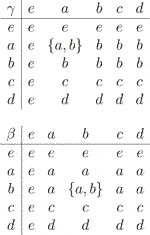Then, S is a $\text{Γ}$-semihypergroup . We have $\left(S,\Gamma ,\le \right)$ as an ordered $\text{Γ}$-semihypergroup, where the order relation $\le$ is defined by

$≤:={(a,a),(b,a),(b,b),(c,c),(d,c),(d,d),(e,e)}.$

The covering relation and the figure of S are given by

$≺={(b,a),(d,c)}.$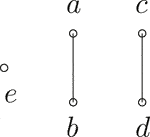Let $\mathrm{ℬ}=\left\{e,a,b\right\}$, ${\mathcal{J}}_{1}=\left\{c\right\}$, and ${\mathcal{J}}_{2}=\left\{d\right\}$. It is easy to see that $\mathrm{ℬ}$ is a $\left({\mathcal{J}}_{1},{\mathcal{J}}_{2}\right)$-bi-$\text{Γ}$-hyperideal of S.

The concept of $\mathcal{J}$-bi-$\text{Γ}$-hyperideals of an ordered $\text{Γ}$-semihypergroup is a generalization of the concept of $\mathcal{J}$-$\text{Γ}$-hyperideals (left $\mathcal{J}$-$\text{Γ}$-hyperideals and right $\mathcal{J}$-$\text{Γ}$-hyperideals) of an ordered $\text{Γ}$-semihypergroup. Obviously, every left (right) $\mathcal{J}$-$\text{Γ}$-hyperideal of an ordered $\text{Γ}$-semihypergroup S is a $\mathcal{J}$-bi-$\text{Γ}$-hyperideal of S, but the following example shows that the converse is not true in the general case.

Example 5. Consider the ordered $\text{Γ}$-semihypergroup $\left(S,\text{Γ},\le \right)$ given in Example 2. It is easy to check that $\mathrm{ℬ}=\left\{a,b,c\right\}$ is a $\mathcal{J}$-bi-$\text{Γ}$-hyperideal on S, where $\mathcal{J}=\left\{b,f\right\}$, but it is not a right $\mathcal{J}$-$\text{Γ}$-hyperideal on S. Since $c\in \mathrm{ℬ}$ and $f\in \mathcal{J}$, but $c\gamma f=\left\{a,f\right\}\mathrm{⊈}\left\{a,b,c\right\}$ which implies that $\mathrm{ℬ}\Gamma \mathcal{J}\subseteq \mathrm{ℬ}$ does not hold.

Theorem 3.11. Let $\left(S,\text{Γ},\le \right)$ be an ordered $\text{Γ}$-semihypergroup and ${\mathcal{J}}_{1}$ and ${\mathcal{J}}_{2}$ be two sub-$\text{Γ}$-semihypergroups of S. Then, S is left ${\mathcal{J}}_{1}$-simple and right ${\mathcal{J}}_{2}$-simple if and only if S does not contain proper $\left({\mathcal{J}}_{1},{\mathcal{J}}_{2}\right)$-bi-$\text{Γ}$-hyperideals.

Proof. Let S be a left ${\mathcal{J}}_{1}$-simple and right ${\mathcal{J}}_{2}$-simple ordered $\text{Γ}$-semihypergroup and B a $\left({\mathcal{J}}_{1},{\mathcal{J}}_{2}\right)$-bi-$\text{Γ}$-hyperideal of S. It is sufficient to prove that $S\subseteq B$. Consider $s\in S$ and $b\in B$. Since S is left ${\mathcal{J}}_{1}$-simple, we obtain

$S=L1(b)=(b∪J1Γb]J1,$

by Lemma 3.5. We need to consider only two cases:

Case 1. Let $s\le b$. As B is $\left({\mathcal{J}}_{1},{\mathcal{J}}_{2}\right)$-bi-$\text{Γ}$-hyperideal, we have $s\in B$.

Case 2. Let $s\le {j}_{1}\gamma b$, for some ${j}_{1}\in {\mathcal{J}}_{1}$ and $\gamma \in \text{Γ}$. By assumption, S is a right ${\mathcal{J}}_{2}$-simple ordered $\text{Γ}$-semihypergroup. Therefore,

$S=R2(b)=(b∪bΓJ2]J2,$

by Lemma 3.5. Since ${j}_{1}\in {\mathcal{J}}_{1}\subseteq S$, we have ${j}_{1}\le b$ or ${j}_{1}\in {\left(b\lambda {j}_{2}\right]}_{{\mathcal{J}}_{2}}$ for some ${j}_{2}\in {\mathcal{J}}_{2}$ and $\lambda \in \text{Γ}$. By Lemma 3.6, we have

$S=(bΓJ2]J=(J1Γb]J,$

and so

$b∈(bΓJ2]J⊆(bΓ(J1Γb]J]J⊆(bΓJ1Γb]J⊆(bΓJΓb]J,$

where $\mathcal{J}={\mathcal{J}}_{1}\cup {\mathcal{J}}_{2}$. Hence, S is a $\mathcal{J}$-regular ordered $\text{Γ}$-semihypergroup. So, there exist $x\in \mathcal{J}$ and $\alpha ,\beta \in \text{Γ}$ such that $b\le b\alpha x\beta b$. We now turn to the case ${j}_{1}\le b$. From this, we conclude that ${j}_{1}\gamma b\le b\gamma b$. So, we obtain

$(j1γb]J⊆(bγb]J⊆(bγ(bαxβb)]J⊆(bγbα(xβb)]J⊆(BΓJΓB]J⊆(B]J=B.$

Since $s\le {j}_{1}\gamma b$, it follows that $s\in B$. This gives $S\subseteq B$. If ${j}_{1}\in {\left(b\lambda {j}_{2}\right]}_{{\mathcal{J}}_{2}}$, then

$(j1γb]J⊆((bλj2]J2γb]J⊆(BΓJΓB]J⊆(B]J=B,$

and so $s\in B$. We thus get $S\subseteq B$.

Conversely, suppose that S does not contain proper $\left({\mathcal{J}}_{1},{\mathcal{J}}_{2}\right)$-bi-$\text{Γ}$-hyperideals. Let M be a left ${\mathcal{J}}_{1}$-$\text{Γ}$-hyperideal and right ${\mathcal{J}}_{2}$-$\text{Γ}$-hyperideal of S. We have

$MΓ(J1∪J2)ΓM=MΓJ1ΓM∪MΓJ2ΓM⊆MΓM⊆M.$

Hence, M is a $\left({\mathcal{J}}_{1},{\mathcal{J}}_{2}\right)$-bi-$\text{Γ}$-hyperideal of S. By assumption, we have $S=M$. Therefore, S is a left ${\mathcal{J}}_{1}$-simple and right ${\mathcal{J}}_{2}$-simple ordered $\text{Γ}$-semihypergroup.

## 4. Relative (Bi)-Int-$\text{Γ}$-Hyperideals ($\mathcal{J}$-(Bi)-Int-$\text{Γ}$-Hyperideals)

Definition 4.1. Let $\left(S,\Gamma ,\le \right)$ be an ordered $\text{Γ}$-semihypergroup and $\mathcal{J}$ a nonempty subset of S. A sub-$\text{Γ}$-semihypergroup ${\mathcal{C}}_{I}$ of S is called a $\mathcal{J}$-int-$\text{Γ}$-hyperideal of S if the following conditions hold:

(1) $\mathcal{J}\Gamma {\mathcal{C}}_{I}\Gamma \mathcal{J}\subseteq {\mathcal{C}}_{I}$

(2) If $x\in \mathcal{J}$, $y\in {\mathcal{C}}_{I}$, and $x\le y$, then $x\in {\mathcal{C}}_{I}$

It is not difficult to see that every $\mathcal{J}$-$\text{Γ}$-hyperideal of an ordered $\text{Γ}$-semihypergroup S is a $\mathcal{J}$-int-$\text{Γ}$-hyperideal of S. The following example shows that the converse is not true in general.

Example 6. Let $S=\left\{a,b,c,d\right\}$ and $\Gamma =\left\{\gamma \right\}$. We define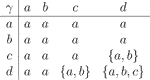Then, S is a $\text{Γ}$-semihypergroup. We have $\left(S,\text{Γ},\le \right)$ is an ordered $\text{Γ}$-semihypergroup, where the order relation $\le$ is defined by

$≤:={(a,a),(a,b),(a,c),(a,d),(b,b),(c,c),(d,d)}.$

The covering relation and the figure of S are given by

$≺={(a,b),(a,c),(a,d)}.$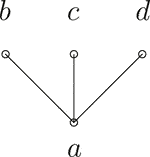Let ${\mathcal{C}}_{I}=\left\{a,c\right\}$ and $\mathcal{J}=\left\{b,d\right\}$. Here, ${\mathcal{C}}_{I}$ is a $\mathcal{J}$-int-$\text{Γ}$-hyperideal of S, but not a $\mathcal{J}$-$\text{Γ}$-hyperideal of S. Indeed, since $\mathcal{J}\Gamma {\mathcal{C}}_{I}=\left\{a,b\right\}\mathrm{⊈}{\mathcal{C}}_{I}$, it follows that ${\mathcal{C}}_{I}$ is not a $\mathcal{J}$-$\text{Γ}$-hyperideal of S.The following example shows that a $\mathcal{J}$-regular ordered $\text{Γ}$-semihypergroup is not regular in general.

Example 7. Consider the $\text{Γ}$-semihypergroup $\left(S,\text{Γ}\right)$ given in Example 6. Let $\le$ be the relation on S defined as follows:

$≤:={(a,a),(b,a),(b,b),(b,c),(c,c),(d,d)}.$

Then, $\left(S,\Gamma ,\le \right)$ is an ordered $\text{Γ}$-semihypergroup. The covering relation and the figure of S are given by:

$≺={(b,a),(b,c)}.$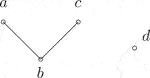Let $\mathcal{J}=\left\{a,b\right\}\subset S$. An easy computation shows that S is a $\mathcal{J}$-regular ordered $\text{Γ}$-semihypergroup, but it is clearly not regular.

Theorem 4.2. Let $\left(S,\Gamma ,\le \right)$ be a $\mathcal{J}$-regular ordered $\Gamma$-semihypergroup, where $\mathcal{J}$ is a nonempty subset of S. Then, every $\mathcal{J}$-int-$\text{Γ}$-hyperideal ${\mathcal{C}}_{I}$$\left({\mathcal{C}}_{I}\subseteq \mathcal{J}\right)$ of S is a $\mathcal{J}$-$\text{Γ}$-hyperideal of S.

Proof. Let ${\mathcal{C}}_{I}$ be a $\mathcal{J}$-int-$\text{Γ}$-hyperideal of S. Then, ${\mathcal{C}}_{I}$ is a sub-$\text{Γ}$-semihypergroup of S and ${\left({\mathcal{C}}_{I}\right]}_{\mathcal{J}}={\mathcal{C}}_{I}$. Let $a\in {\mathcal{C}}_{I}\subseteq \mathcal{J}$. Since S is $\mathcal{J}$-regular, there exist $x\in \mathcal{J}$ and $\alpha ,\beta \in \Gamma$ such that $a\le a\alpha x\beta a$. Now, let $j\in \mathcal{J}$ and $\gamma \in \Gamma$. Then,

$jγa≤jγ(aαxβa)=(jγaαx)βa⊆(JΓCIΓJ)ΓCI⊆CIΓCI⊆CI.$

Thus, $\mathcal{J}\text{Γ}{\mathcal{C}}_{I}\subseteq {\left({\mathcal{C}}_{I}\right]}_{\mathcal{J}}={\mathcal{C}}_{I}$. By a similar argument, we have ${\mathcal{C}}_{I}\text{Γ}\mathcal{J}\subseteq {\mathcal{C}}_{I}$. Hence, the result follows.In the following, we introduce the notion of $\mathcal{J}$-bi-int-$\text{Γ}$-hyperideals as a generalization of $\mathcal{J}$-$\text{Γ}$-hyperideals, $\mathcal{J}$-bi-$\text{Γ}$-hyperideals, and $\mathcal{J}$-int-$\text{Γ}$-hyperideals of ordered $\text{Γ}$-semihypergroups.

Definition 4.3. Let $\left(S,\text{Γ},\le \right)$ be an ordered $\text{Γ}$-semihypergroup and $\mathcal{J}$ a nonempty subset of S. A sub-$\text{Γ}$-semihypergroup ${\mathcal{D}}_{b-i}$ of S is called a $\mathcal{J}$-bi-int-$\text{Γ}$-hyperideal of S if the following conditions hold:

(1) $\mathcal{J}\Gamma {\mathcal{D}}_{b-i}\Gamma \mathcal{J}\cap {\mathcal{D}}_{b-i}\Gamma \mathcal{J}\Gamma {\mathcal{D}}_{b-i}\subseteq {\mathcal{D}}_{b-i}$

(2) If $x\in \mathcal{J}$, $y\in {\mathcal{D}}_{b-i}$, and $x\le y$, then $x\in {\mathcal{D}}_{b-i}$

Lemma 4.4. Let $\left(S,\text{Γ},\le \right)$ be an ordered $\text{Γ}$-semihypergroup and $\mathcal{J}$ a nonempty subset of S. Then, the following statements hold:

(1) Every $\mathcal{J}$-$\text{Γ}$-hyperideal of S is a $\mathcal{J}$-bi-int-$\text{Γ}$-hyperideal of S

(2) The intersection of $\mathcal{J}$-bi-int-$\text{Γ}$-hyperideals of S is a $\mathcal{J}$-bi-int-$\text{Γ}$-hyperideal of S

(3) If B is a $\mathcal{J}$-bi-$\text{Γ}$-hyperideal and C a $\mathcal{J}$-int-$\text{Γ}$-hyperideal of S, then ${\mathcal{D}}_{b-i}=B\cap C$ is a $\mathcal{J}$-bi-int-$\Gamma$-hyperideal of S

Proof. (1) Let A be a $\mathcal{J}$-$\text{Γ}$-hyperideal of S. Then, $\mathcal{J}\Gamma A\subseteq A$ and $A\Gamma \mathcal{J}\subseteq A$. We have

$JΓAΓJ∪AΓJΓA⊆AΓJΓA⊆AΓA⊆A,$

and

$JΓAΓJ∪AΓJΓA⊆JΓAΓJ⊆AΓJ⊆A.$

Therefore, A is a $\mathcal{J}$-bi-int-$\text{Γ}$-hyperideal of S.

(2) The proof is similar to the proof of Lemma 3.8.

(3) Clearly, ${\mathcal{D}}_{b-i}=B\cap C$ is a sub-$\text{Γ}$-semihypergroup of S. We have

$Db−iΓJΓDb−i=(B∩C)ΓJΓ(B∩C)⊆BΓJΓB⊆B,$

and

$JΓDb−iΓJ=JΓ(B∩C)ΓJ⊆JΓCΓJ⊆C.$

Hence, $\mathcal{J}\Gamma {\mathcal{D}}_{b-i}\Gamma \mathcal{J}\cap {\mathcal{D}}_{b-i}\Gamma \mathcal{J}\Gamma {\mathcal{D}}_{b-i}\subseteq B\cap C={\mathcal{D}}_{b-i}$. Therefore, ${\mathcal{D}}_{b-i}=B\cap C$ is a $\mathcal{J}$-bi-int-$\text{Γ}$-hyperideal of S.

Example 8. Let $S=\left\{a,b,c,d,e\right\}$ and $\Gamma =\left\{\gamma \right\}$. We define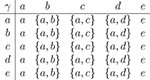Then, S is a $\text{Γ}$-semihypergroup. We have $\left(S,\Gamma ,\le \right)$ as an ordered $\text{Γ}$-semihypergroup , where the order relation $\le$ is defined by

$≤:={(a,a),(b,b),(c,c),(d,d),(e,e),(a,c),(b,a),(b,c),(d,a),(d,c),(e,a),(e,c)}.$

The covering relation and the figure of S are given by

$≺={(a,c),(b,a),(d,a),(e,a)}.$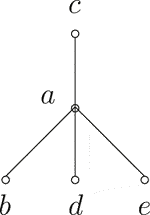Let ${\mathcal{D}}_{b-i}=\left\{a,b,d\right\}$ and $\mathcal{J}=\left\{c,d\right\}$. Then, $\mathcal{J}\Gamma {\mathcal{D}}_{b-i}\Gamma \mathcal{J}=\left\{a,c,d\right\}$ and ${\mathcal{D}}_{b-i}\Gamma \mathcal{J}\Gamma {\mathcal{D}}_{b-i}=\left\{a,b,d\right\}$, so $\left\{a,c,d\right\}\cap \left\{a,b,d\right\}=\left\{a,d\right\}\subseteq {\mathcal{D}}_{b-i}$. Hence, ${\mathcal{D}}_{b-i}$ is a $\mathcal{J}$-bi-int-$\text{Γ}$-hyperideal of S. It is easy to see that ${\mathcal{D}}_{b-i}$ (${\mathcal{D}}_{b-i}\Gamma \mathcal{J}\mathrm{⊈}{\mathcal{D}}_{b-i}$) is not a $\mathcal{J}$-$\text{Γ}$-hyperideal of S.

## 5. Conclusion

In this article, we studied some properties of $\mathcal{J}$-(bi-)$\text{Γ}$-hyperideals of ordered $\text{Γ}$-semihypergroups. In particular, we introduced and studied $\mathcal{J}$-int-$\text{Γ}$-hyperideals and $\mathcal{J}$-bi-int-$\text{Γ}$-hyperideals. Furthermore, we proved that $\mathcal{J}$-int-$\text{Γ}$-hyperideals and $\mathcal{J}$-$\text{Γ}$-hyperideals coincide in $\mathcal{J}$-regular ordered $\text{Γ}$-semihypergroups. When we deal with $\mathcal{J}$-(bi-)$\text{Γ}$-hyperideals of ordered $\text{Γ}$-semihypergroups, it is natural to talk about fuzzy $\mathcal{J}$-(bi-)$\text{Γ}$-hyperideal. According to the research results, it is suggested to define and investigate some properties of fuzzy $\mathcal{J}$-(bi-)$\text{Γ}$-hyperideals, rough prime $\mathcal{J}$-bi-$\text{Γ}$-hyperideals, fuzzy prime $\mathcal{J}$-bi-$\text{Γ}$-hyperideals, and uni-soft $\mathcal{J}$-int-$\text{Γ}$-hyperideals in ordered $\text{Γ}$-semihypergroups. As an application of the results of this article, the corresponding results of ordered semihypergroups can be also obtained by moderate modification.

## Data Availability Statement

All datasets presented in this study are included in the article/supplementary material.

## Author Contributions

All authors have made a substantial, direct, and intellectual contribution to the work and approved it for publication.

## Funding

This work was supported by the National Key R&D Program of China (Grant no. 2019YFA0706402), the Natural Science Foundation of Guangdong Province (Grant no. 2018A0303130115), and Guangzhou Academician and Expert Workstation (Grant no. 20200115-9).

## Conflict of Interest

The authors declare that the research was conducted in the absence of any commercial or financial relationships that could be construed as a potential conflict of interest.

## References

1. Dehghan Nezhad, A, Moosavi Nejad, SM, Nadjafikhah, M, and Davvaz, B. A physical example of algebraic hyperstructures: leptons. Indian J Phys. (2012). 86(11):1027–32. doi: 10.1007/s12648-012-0151-x

2. Davvaz, B, Nezhad, AD, and Nejad, SMM. Algebraic hyperstructure of observable elementary particles including the Higgs boson. Proc Natl Acad Sci, India, Sect A Phys Sci. (2020). 90:169–76. doi: 10.1007/s40010-018-0553-z

3. Yaqoob, N, Tang, J, and Chinram, R. Structures of involution Γ-semihypergroups. Honam Mathematical J (2018). 40(1):109–24. doi: 10.5831/HMJ.2018.40.1.109

4. Khan, A, Farooq, M, and Yaqoob, N. Uni-soft structures applied to ordered Γ-semihypergroups. Proc Natl Acad Sci, India, Sect A Phys Sci. (2020). 90:457. doi: 10.1007/s40010-019-00602-x

5. Marty, F. Sur une generalization de la notion de groupe. In: Proceedings of the 8th Congress Math. Scandinaves, Stockholm, Sweden; Stockholm, Sweden (1934). p. 45–49.

6. Corsini, P. Prolegomena of hypergroup theory. 2nd ed. Udine, Italy: Aviani Editore (1993).

7. Corsini, P, and Leoreanu, V. Applications of hyperstructure theory. In: Advances in mathematics. Dordrecht, Netherlands: Kluwer Academic Publishers (2003).

8. Davvaz, B. Semihypergroup theory. New York, NY: Elsevier (2016).

9. Davvaz, B, and Leoreanu-Fotea, V. Hyperring theory and applications. Palm Harbor, FL: International Academic Press (2007).

10. Davvaz, B, and Vougiouklis, T. A walk through weak hyperstructures-Hυ-structures. Hackensack, NJ: World Scientific Publishing Co. Pte. Ltd. (2019).

11. Vougiouklis, T. Hyperstructures and their representations. Palm Harbor, FL: Hadronic Press Inc. (1994).

12. Heidari, D, and Davvaz, B. On ordered hyperstructures. Politehn Univ Bucharest Sci Bull Ser A Appl Math Phys. (2011). 73(2):85–96.

13. Davvaz, B, Corsini, P, and Changphas, T. Relationship between ordered semihypergroups and ordered semigroups by using pseudoorder. Eur J Combinator. (2015). 44:208–17. doi: 10.1016/j.ejc.2014.08.006

14. Gu, Z, and Tang, X. Ordered regular equivalence relations on ordered semihypergroups. J Algebra. (2016). 450:384–97. doi: 10.1016/j.jalgebra.2015.11.026

15. Tipachot, N, and Pibaljommee, B. Fuzzy interior hyperideals in ordered semihypergroups. Ital J Pure Appl Math. (2016). 36:859–70.

16. Mahboob, A, Khan, NM, and Davvaz, B. (m, n)-hyperideals in ordered semihypergroups. Categ General Alg Structures Appl. (2020). 12(1):43–67. doi: 10.29252/cgasa.12.1.43

17. Good, RA, and Hughes, DR. Associated groups for a semigroup. Bull Am Math Soc. (1952). 58:624–5.

18. Wallace, A. Relative ideals in semigroups, I (Faucett’s theorem). Colloq Math. (1962). 9:55–61. doi: 10.4064/cm-9-1-55-61.

19. Hrmová, R. Relative ideals in semigroups. Matematický časopis (1967). 17(3):206–23.

20. Khan, NM, and Ali, MF. Relative bi-ideals and relative quasi ideals in ordered semigroups. Hacet J Math Stat (2020). 49(3):950–61. doi: 10.15672/hujms.624046

21. Sen, MK, and Saha, NK. On Γ-semigroup I. Bull Calcutta Math Soc. (1986). 78:180–6.

22. Anvariyeh, SM, Mirvakili, S, and Davvaz, B. On Γ-hyperideals in Γ-semihypergroups. Carpathian J Math. (2010). 26(1):11–23.

23. Yaqoob, N, and Aslam, M. Prime (m, n) Bi-Γ -hyperideals in Γ-semihypergroups. Appl Math Inf Sci. (2014). 8(5):2243–9. doi: 10.12785/amis/080519

24. Omidi, S, and Davvaz, B. Bi-Γ-hyperideals and Green’s relations in ordered Γ-semihypergroups. Eurasian Mathematical J (2017). 8(4):63–73.

25. Omidi, S, Davvaz, B, and Hila, K. Characterizations of regular and intra-regular ordered Γ-semihypergroups in terms of bi-Γ-hyperideals. Carpathian Math Publ. (2019). 11(1):136–51. doi: 10.15330/cmp.11.1.136-151

26. Basar, A, Abbasi, MY, and Bhavanari, S. On generalized Γ-hyperideals in ordered Γ-semihypergroups. Fundam J Math Appl. (2019). 2(1):18–23. doi: 10.33401/fujma.543712

27. Tang, J, Davvaz, B, Xie, X-Y, and Yaqoob, N. On fuzzy interior Γ-hyperideals in ordered Γ-semihypergroups. J Intell Fuzzy Syst. (2017). 32:2447–60. doi: 10.3233/jifs-16431

28. Omidi, S, Davvaz, B, and Abdiogluv, C. Some properties of quasi-Γ-hyperideals and hyperfilters in ordered Γ-semihypergroups. Southeast Asian Bull Math. (2018). 42(2):223–42.

29. Omidi, S, and Davvaz, B. Convex ordered Gamma-semihypergroups associated to strongly regular relations. Matematika (2017). 33(2):227–40. doi: 10.11113/matematika.v33.n2.838

Keywords: ordered$\text{⌈}$ -semihypergroup, $\mathcal{J}$ -bi- $\text{⌈}$ -hyperideal, $\mathcal{J}$ -(bi-)int- $\text{⌈}$ -hyperideal, $\mathcal{J}$ -regular AMS mathematics subject classification: 16Y99, 20N20, 06F99

Citation: Rao Y, Xu P, Shao Z, Kosari S and Omidi S (2020) Some Properties of Relative Bi-(Int-)Γ-Hyperideals in Ordered Γ-Semihypergroups. Front. Phys. 8:555573. doi: 10.3389/fphy.2020.555573

Received: 25 April 2020; Accepted: 24 August 2020;
Published: 05 November 2020.

Edited by:

Muhammad Javaid, University of Management and Technology, Lahore, Pakistan

Reviewed by:

Kazuharu Bamba, Fukushima University, Japan
Naveed Yaqoob, Majmaah University, Saudi Arabia

Copyright © 2020 Rao, Xu, Shao, Kosari and Omidi. This is an open-access article distributed under the terms of the Creative Commons Attribution License (CC BY). The use, distribution or reproduction in other forums is permitted, provided the original author(s) and the copyright owner(s) are credited and that the original publication in this journal is cited, in accordance with accepted academic practice. No use, distribution or reproduction is permitted which does not comply with these terms.

*Correspondence: S. Kosari, saeedkosari38@yahoo.com